# AP Board 4th Class Maths Solutions 4th Lesson Subtraction

Andhra Pradesh AP Board 4th Class Maths Solutions 4th Lesson Subtraction Textbook Exercise Questions and Answers.

## AP State Syllabus 4th Class Maths Solutions Chapter 4 Subtraction

Textbook Page No. 47

Do this

Question 1.
a)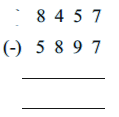Solution: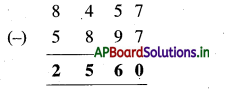b)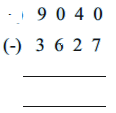Solution: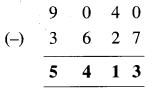c)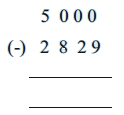Solution: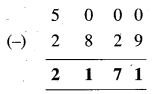Question 2.
Subtract 4,385 from 9,230
Solution: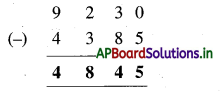Textbook Page No. 48

Do this

Question 1.
Sri Krishna had ₹ 9,213 in his bank account. He withdrew ₹ 7,435. How much money was left in his account ?
Solution:
Amount in Krishna’s bank account = ₹ 9,213
Withdrew amount = ₹ 7,435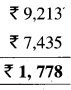Left amount in his account = ₹ 1, 778Question 2.
Nanaji wanted to buy a sprayer of cost 9,500. Government provided a subsidy of 2,500. Then how much money should he pay ?
Solution:
Cost of Sprayer = ₹ 9,500
Amount of subsidy provided = ₹ 2,500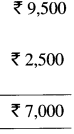Remaining amount should be pay = ₹ 7,000

Textbook Page No. 49

Do this

Question 1.
In a village, there are 8142 trees. Out of these 3780 trees fell down due to Hud-hud cyclone. The number of trees remaining is ………….
(Approximately)
a) 3,000
b) 4,000
c) 5,000
d) 6,000
Solution:
[b]
8,000 – 4,000 = 4,000

Question 2.
The sum of two numbers is 7,152 and one of the numbers is 5,200. The other number nearest to thousand is
Solution:
7.000 – 5,000 = 2,000Question 3.
Write correct symbol of <, >, or = by estimating the difference.
a) 2,300 – 800        <              2,950 – 1100
b) 4,100 – 1,800             =            8,005 – 6,200
c) 3,900 – 890           >           7,020 – 5,638

Textbook Page No. 49

Try this

Correct the following subtractions.

a)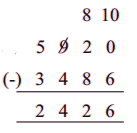Solution:
Correct Subtraction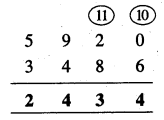b)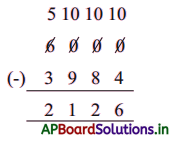Solution:
Correct Subtraction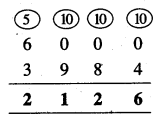Textbook Page No. 50

Do this

1. Subtract mentally.

a) 95 – 21
Solution:
Clearly 21 is 20 + 1
95 – 21 =95 – 20 – 1
= 75 – 1
= 74

b) 88 – 55
Solution:
Clearly 55 is 50 + 5
88 – 50 = 38 – 5
= 33

c) 68 – 47
Solution:
Clearly 47 is 50 – 3
68 – 50 = 18 + 3
= 21

d) 52 – 26
Solution:
Clearly 26 is 30 – 4
52 – 30 = 22 + 4
= 26e) 73 – 37
Solution:
Clearly 37 is 40 – 3
73 – 40 = 33 + 3
= 36

2. Write subtraction facts for the given addition facts.

a) 734 + 268 = 1002
Solution:
734 + 268 = 1002
1002 – 268 = 734

b) 3,140 + 2,869 = 6,009
Solution:
3,140 + 2,869 = 6,009
6009 – 2,869 = 3,140
We write subtraction fact from given addition fact.

3. Write addition facts for the given subtraction facts.

a) 480 – 320 = 160
Solution:
Given subtraction is
480 – 320 = 160
160 + 320 = 480
We write addition fact for a subtraction fact.b) 5,286 – 3,812 = 1,474
Solution:
Given subtraction is
5,286 – 3,812 = 1,474
5,286 = 1,474 + 3,812
We write addition fact for a subtraction fact

Exercise – 4.1

1. Do the following.

a)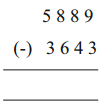Solution: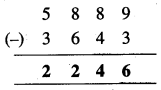b)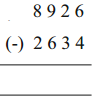Solution: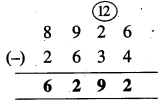c)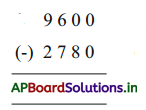Solution: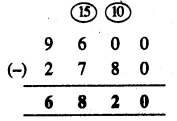d)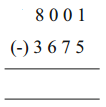Solution: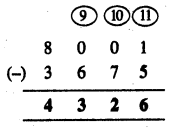e)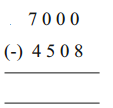Solution: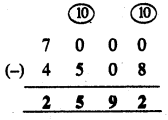Question 2.
Subtract 7,425 from 9,015.
Solution: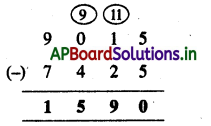Question 3.
Take away 3086 from 8,415
Solution: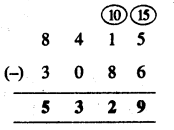Question 4.
By how much is 3,189 more than 2883?
Solution:
3,189 – 2883 = 306
∴ 3,189 is 306 more than 2,883.

Question 5.
Estimate the difference to the nearest thousands by rounding.
Solution:
5,742 – 4,265 = 6,000 – 4000
= 2,000

Question 6.
Do a quick estimate to check which of the following is more than 4,000.
a) 5555 – 1266
Solution:
6,000 – 1,000 = 5,000

b) 9885 – 7657
Solution:
10,000 – 8,000 = 2,000

Question 7.
Fill the place holders with <, > or =
a) 5,000 – 1,200          >           3,600 – 2,400
b) 9,200 – 4,020          <            7,680 – 2118
c) 7,900 – 4,200             =           6,020 – 1,950Question 8.
In a school, the children collected 8562 for CM Relief Fund. The staff donated 2892 less than the amount donated by children. How much money did the staff donate ?
Solution:
Amount collected by children = 8,562
Staff donated 2892 less than the amount donated by children.
∴ Money donated by the staff = 8562 – 2892
= 5,670

Question 9.
A website was visited by 9,125 people on its first day and 6,532 people on the second day. How many more people visited the website on the first day than on the second day ?
Solution:
Number of people visited the website on first day = 9125
Number of people visited the website on second day = 6532
Difference = 9125 – 6532
= 2,593
2,593 people visited the website on the first day than on the second day.

Question 10.
Abhiram covered a journey of 3120 kilometres to Kashmeer. He travelled 1,968 kilometres by train and the rest by bus. What distance did he covered by bus?
Solution:
Actual distance covered by Abhiram = 3120 km
Abhiram travelled distance by train = 1968
Abhiram travelled distance by bus
= 3120-1968
= 1152km

Textbook Page No. 55

Do this

Write the Cost price and Selling Price from the following contexts.
a) Seetha bought lemons for ₹ 600 and sold them for ₹ 850.
Solution:
Cost price of lemon = ₹ 600
Selling price of lemon = ₹ 850b) Laxmi bought flowers for ₹ 1,500 and sold them for ₹ 1,350.
Solution:
Cost price of flowers = ₹ 1,500
Selling price of flowers = ₹ 1,300

c) Veerayya sold bananas for ₹ 2,450 and previously he bought for ₹ 1,940.
Solution:
Cost price of bananas = ₹ 1,940
Selling price of bananas = ₹ 2,450

d) Adilaxmi bought leafy vegetables for ₹ 150 and sold them for ₹ 120.
Solution:
Cost price of leafy vegetables = ₹ 150
Selling price of leafy vegetables = ₹ 120

Complete the table :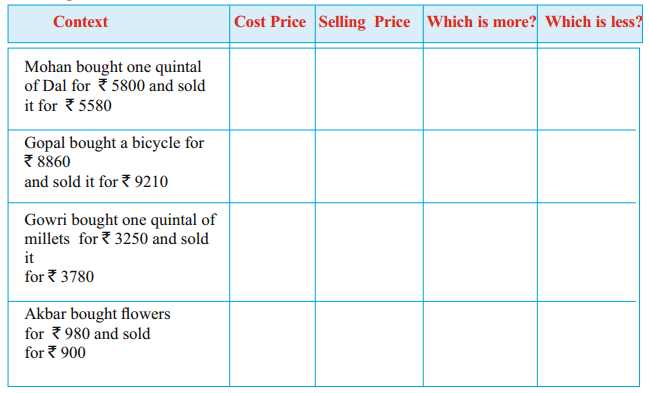Solution: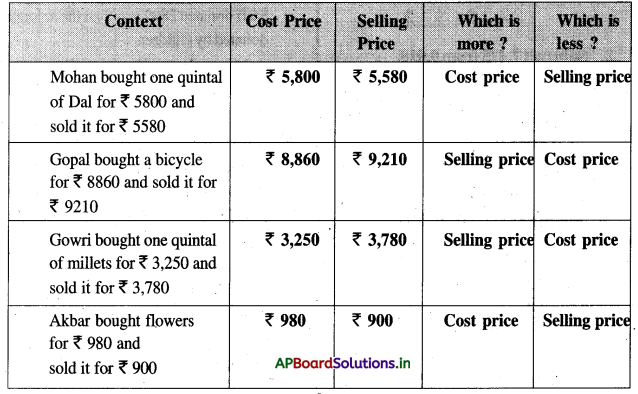Textbook Page No. 55

Do this

1. Say whether profit or loss made in each of the following cases.

a) CP = ₹ 3,100
SP = ₹ 2950
Solution:
C.P < S.P.
So loss made in this case.

b) CP = ₹ 2,505 SP = ₹ 3,160
Solution:
S.P > C.P
So Profit made in this case.Question 2.
Padmaja bought a saree for ₹ 7,500 and sold it to Rupa for 5,850. Say whether Padmaja made profit or loss ?
Solution:
C.P. > S.P.

Exercise – 4.2

1. Write ‘P’ for Profit and ‘L’ for Loss in the given brackets for the following.

a) CP = ₹ 420
SP = ₹ 390
Solution:
L

b) CP = ₹ 920
SP = ₹ 990
Solution:
P

c) CP = ₹ 4860
SP = ₹ 5002
Solution:
F

d) CP = ₹ 3140
SP = ₹ 2849
Solution:
Le) CP = ₹ 2195
SP = ₹ 3000
Solution:
P

Question 2.
A shop keeper bought a bag of sugar for ₹ 1650 and sold it for ₹ 90 more. Say whether he made profit or loss ?
Solution:
Cost price of bag of sugar = ₹ 1650
Selling price is ₹ 90 more than cost price
Selling price of bag of sugar
= 1650 + 90 = ₹ 1740
S.P > C.P

Question 3.
Kumar bought oranges for ₹ 1520 and sold them for ₹ 150 less. Say whether it is profit or loss ?
Solution:
Cost price of oranges = ₹ 1520
Selling price is ₹ 150 less than cost price.
Selling price of oranges = 1,520 – 150
= ₹ 4,370
C.P > S.P

Question 4.
Rahim bought umbrellas for ₹ 2100 and sold ₹ 1950. Say whether it is profit or loss?
Solution:
Cost price of umbrellas = ₹ 2100
Selling price of umbreallas = ₹ 1950
C.P < S.P.Question 5.
Salman bought a goat for ₹ 7,850 and sold it for ₹ 8,325. Say whether it is profit or loss?
Solution:
Cost price of goat = ₹ 7,850
Selling price of goat = ₹ 8325
S.P > C.P.

Multiple Choice Questions

Question 1.
In subtraction greater number is called
A) Subtrahend
B) Minuend
C) Difference
D) None
B) Minuend

Question 2.
In subtraction less number is called
A) Subtrahend
B) Minuend
C) Difference
D) None
A) SubtrahendQuestion 3.
Result between Minuend subtrahend is
A) Subtrahend
B) Minuend
C) Difference
D) None
C) Difference

Question 4.
In 9,467 – 4,235 = 5232, Difference is
A) 9,467
B) 4,235
C) 5,232
D) None
C) 5,232

Question 5.
The difference between 8142 and 4780 nearly thousands
A) 2,000
B) 3,000
C) 4,000
D) 5,000
B) 3,000Question 6.
Complete the pattern 30, 40, 50……………
A) 70
B) 60
C) 80
D) 90
B) 60

Question 7.
The difference between 368 and 215 is nearly hundreds
A) 100
B) 200
C) 300
D) 400
B) 200

Question 8.
Choose the Kaprekar number from options
A) 1467
B) 6174
C) 7164
D)4167
B) 6174

Question 9.
The amount of money which we pay to buy an article is called
A) Cost price
B) Selling price
C) Profit
D) Loss
A) Cost priceQuestion 10.
The amount which we receive from a customer is called
A) Cost price
B) Selling price
C) Profit
D)Loss
Solution:
B) Selling price

Question 11.
C.P > S.P then merchant faces
A) Cost price
B) Selling price
C) Profit
D) Loss